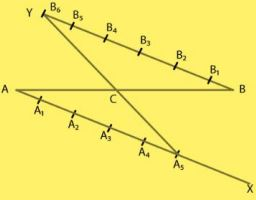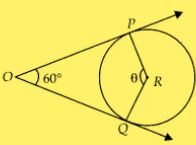# Chapter 10 Construction NCERT Exemplar Solutions Exercise 10.1 Class 10 MathsChapter Name NCERT Maths Exemplar Solutions for Chapter 10 Construction Exercise 10.1 Book Name NCERT Exemplar for Class 10 Maths Other Exercises Exercise 10.2Exercise 10.3Exercise 10.4 Related Study NCERT Solutions for Class 10 Maths

### Exercise 10.1 Solutions

Multiple Choice Questions

Choose the correct answer from the given four options:

1. To divide a line segment AB in the ratio 5:7, first a ray AX is drawn so that ∠BAX is an acute angle and then at equal distances points are marked on the ray AX such that the minimum number of these points is
(A) 8
(B) 10
(C) 11
(D) 12
Solution
(D) 12
As given in the question,
A line segment AB in the ratio 5:7
So,
A : B = 5:7
We draw a ray AX making an acute angle ∠BAX,
And mark A+B points at equal distance.
A = 5 and B = 7
Therefore,
Minimum number of these points = A + B
= 5 + 7 = 12

2. To divide a line segment AB in the ratio 4:7, a ray AX is drawn first such that BAX is an acute angle and then points A1 , A2 , A3 ... are located at equal distances on the ray AX and the point B is joined to
(A) A12
(B) A11
(C) A10
(D) A9
Solution
(B) A11
As given in the question,
A line segment AB in the ratio 4:7
So,
A:B = 4:7
Now,
Draw a ray AX making an acute angle BAX
Minimum number of points located at equal distances on the ray,
AX = A+B
= 4+7
= 11
A1 , A2 , A3 ... are located at equal distances on the ray AX.
Point B is joined to the last point is A11 .

3.To divide a line segment AB in the ratio 5 : 6, draw a ray AX such that ∠BAX is an acute angle, then draw a ray BY parallel to AX and the points A1 , A2 , A1 , ... and B1 , B2 , B3 , ... are located at equal distances on ray AX and BY, respectively. Then the points joined are
(A) A5 and B6
(B) A6 and B5
(C) A4 and B5
(D) A5 and B4
Solution
(A) A5 and B6
As given in the question,
A line segment AB in the ratio 5:7
So,
A:B = 5:7
Steps of construction:
1. Draw a ray AX, an acute angle BAX.
2. Draw a ray BY ||AX, angle ABY = angle BAX.
3. Now, locate the points A1 , A2 , A3 , A4 ...  and A5 on AX and B1 ,B2 ,B3 ,B4 ,B5  and B6
(Because A: B = 5:7)
4. 4. Join A5B6 .
A5B6 intersect AB at a point C.
AC: BC= 5:64. To construct a triangle similar to a given ΔABC with its sides 3/7 of the corresponding sides of ΔABC, first draw a ray BX such that ∠CBX is an acute angle and X lies on the opposite side of A with respect to BC. Then locate points B1 , B2 , B3 , ... on BX at equal distance and next step is to join
(A) B10  to C
(B) B3 to C
(C) B7 to C
(D) B4 to C
Solution
(C)
In this, we locate points B1 ,B2 ,B3 ,B4 ,B5 , B6  and B7  on BX at equal distance and in next step
join the last point B7  to C.

5. To construct a triangle similar to a given ΔABC with its sides 8/5 of the corresponding sides of ΔABC draw a ray BX such that ∠CBX is an acute angle and X is on the opposite side of A with respect to BC. The minimum number of points to be located at equal distances on ray BX is
(A) 5
(B) 8
(C) 13
(D) 3
Solution
(B)
To construct a triangle similar to a given triangle, with its sides m/n of the n corresponding sides of given triangle the minimum number of points to be located at equal distance is equal to the greater of m and n in m/n . Here,  m/n = 8/5 So, the minimum number of point to be located at equal distance on ray BX is 8.

6. To draw a pair of tangents to a circle which are inclined to each other at an angle of 60°, it is required to draw tangents at end points of those two radii of the circle, the angle between them should be
(A) 135°
(B) 90°
(C) 60°
(D) 120°
Solution
(D)
The angle between them should be 120° because in that case the figure formed by the
intersection point of pair of tangent, the two end points of those two radii (at which tangents
are drawn) and the centre of the circle is a quadrilateral.From figure POQR is a quadrilateral,
∠POQ + ∠PRQ = 180°  [as, sum of opposite angles are 180°]
⇒ 60° + θ = 180°
⇒ θ = 120°
Therefore, the required angle between them is 120.